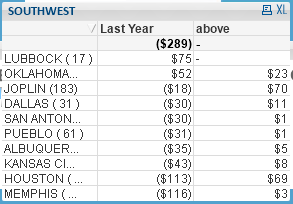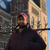Announcements
MAINTENANCE ALERT: Dec. 12th starting 9 AM CET. Community will be read-only. GET DETAILS
cancel
Showing results for
Did you mean:Creator

I need to show "sales from the lead".  It's like in golf where they have "strokes from the lead".  I tried the above function and several variations, but it isn't giving me what I need.  So, below is the sales growth and then next to it, the above formula.

my growth formula is as follows:

sum({<Year={\$(=Max(Year))}, VendId={'100423', '100047', '100060'}, Month={'Sep','Oct','Nov','Dec'}, CurPrevYTD={1},Whse_District={'SOUTHWEST'}>}  NetSalesDOM)/1000
-
sum({<Year={\$(=Max(Year)-1)}, VendId={'100423', '100047', '100060'}, Month={'Sep','Oct','Nov','Dec'}, CurPrevYTD={1},Whse_District={'SOUTHWEST'}>}  NetSalesDOM)/1000

The first value (\$23) is correct, but the next one should be \$93 and the next should be approx. \$105 and so forth.  It is telling how much the branch needs to sell in order to get up to the leader.1 Solution

Accepted SolutionsChampion

I got it

try this (if you use absolute values for negative values)

=top(sum(your_field))+sum(your_field)

or this if you use your negative values:

=top(sum(your_field))-sum(your_field)

4 RepliesChampion

Hi,

for the first value 23, it is calculated this way: 75-52

for the next expected values, how it is supposed to be calculated ?Creator
Author

Yes, the first one is 75-52.  The next one should be 75-(-18). Or it could be 23+70.  I tried using the above() formula to get the first value and then add the one above it, but that didn't work either...Champion

I got it

try this (if you use absolute values for negative values)

=top(sum(your_field))+sum(your_field)

or this if you use your negative values:

=top(sum(your_field))-sum(your_field)Creator
Author

Thank you!  That works!Community Browser